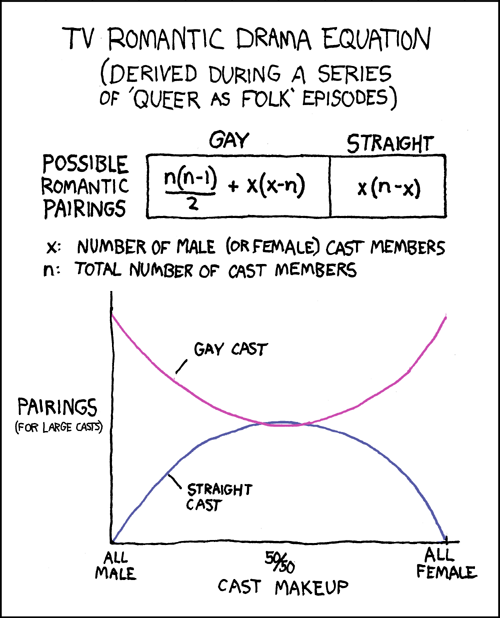← Back

January 29, 2007

# #216: Romantic Drama EquationTV Romantic Drama Equation

(Derived during a series of ‘Queer as Folk’ episodes)

[[A table shows equations for possible romantic pairings in a TV show. The equation under “gay” is n(n-1)

2+x(x-n); the equation under “straight” is x(n-x).]]

x: Number of male (or female) cast members.

n: total number of cast members.

[[A graph plots pairings (for large casts) against cast makeup. Each of the above equations forms a curve.

“Gay cast” starts high for an all male cast, dips down at 50

50 cast makeup, and then rises again for all female.

“Straight cast” starts at zero for an all male cast, peaks at 50

50 cast makeup, and then drops to zero again for an all female cast.

The two curves intersect at two points close to the middle.]]